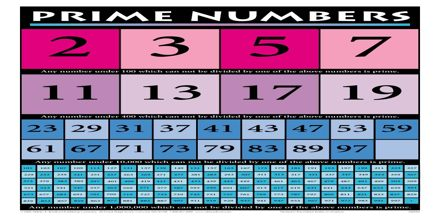Mathematic

# Prime NumbersGeneral purpose of this lecture is to present on Prime Numbers. A natural number (i.e. 1, 2, 3, 4, 5, 6, etc.) is called a prime number (or a prime) if it has exactly two positive divisors, 1 and the number itself. Even Numbers – Any number that can be divided by 2. Odd Numbers – Any number that cannot be divided by 2. Composite Number – An integer that can be divided by at least one other number (a factor) other than itself. Prime Number – An integer whose only factors are 1 and itself. Factor – a number that can divide another number without a remainder.